Aman Gupta — Published On October 10, 2020 and Last Modified On November 30th, 2022

## Introduction

When building a machine learning model in real-life, it’s almost rare that all the variables in the dataset are useful to build a model. Adding redundant variables reduces the generalization capability of the model and may also reduce the overall accuracy of a classifier. Furthermore adding more and more variables to a model increases the overall complexity of the model.

As per the Law of Parsimony of ‘Occam’s Razor’, the best explanation to a problem is that which involves the fewest possible assumptions. Thus, feature selection becomes an indispensable part of building machine learning models.

## Goal

The goal of feature selection techniques in machine learning is to find the best set of features that allows one to build useful models of studied phenomena.

The techniques for feature selection in machine learning can be broadly classified into the following categories:

Supervised Techniques: These techniques can be used for labeled data, and are used to identify the relevant features for increasing the efficiency of supervised models like classification and regression.

Unsupervised Techniques: These techniques can be used for unlabeled data.

From a taxonomic point of view, these techniques are classified as under:

A. Filter methods

B. Wrapper methods

C. Embedded methods

D. Hybrid methods

In this article, we will discuss some popular feature selection techniques in machine learning and feature selection methods in machine learning.

### A. Filter methods

Filter methods pick up the intrinsic properties of the features measured via univariate statistics instead of cross-validation performance. These methods are faster and less computationally expensive than wrapper methods. When dealing with high-dimensional data, it is computationally cheaper to use filter methods.

Let’s, discuss some of these techniques:

Information Gain

Information gain calculates the reduction in entropy from the transformation of a dataset. It can be used for feature selection by evaluating the Information gain of each variable in the context of the target variable.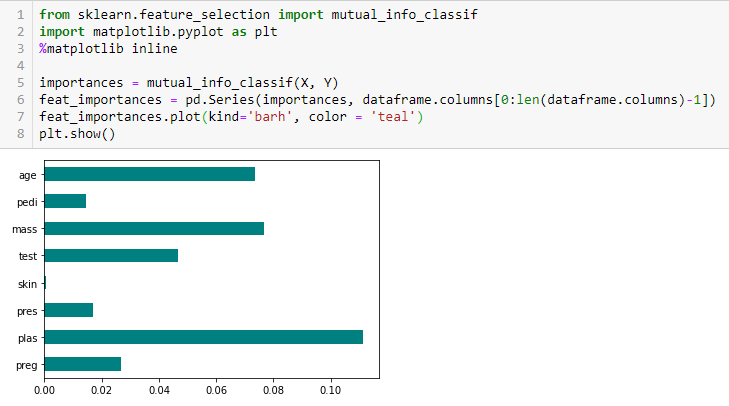#### Chi-square Test

The Chi-square test is used for categorical features in a dataset. We calculate Chi-square between each feature and the target and select the desired number of features with the best Chi-square scores. In order to correctly apply the chi-squared in order to test the relation between various features in the dataset and the target variable, the following conditions have to be met: the variables have to be categorical, sampled independently and values should have an expected frequency greater than 5.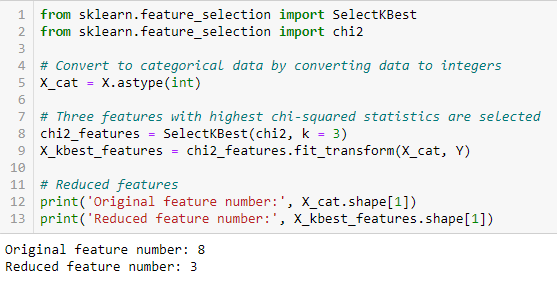#### Fisher’s Score

Fisher score is one of the most widely used supervised feature selection methods. The algorithm which we will use returns the ranks of the variables based on the fisher’s score in descending order. We can then select the variables as per the case.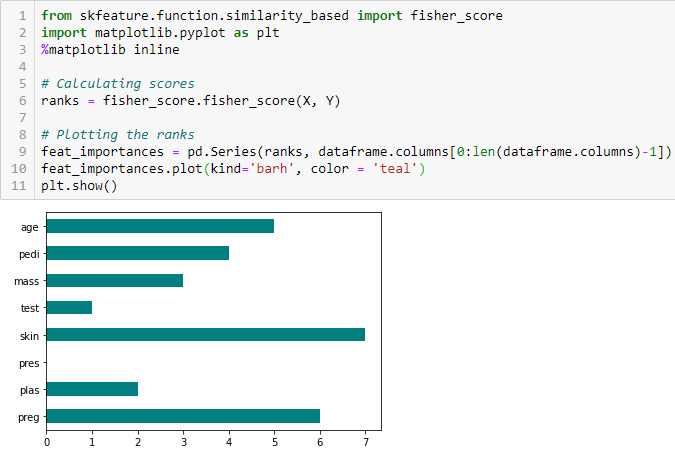#### Correlation Coefficient

Correlation is a measure of the linear relationship of 2 or more variables. Through correlation, we can predict one variable from the other. The logic behind using correlation for feature selection is that the good variables are highly correlated with the target. Furthermore, variables should be correlated with the target but should be uncorrelated among themselves.

If two variables are correlated, we can predict one from the other. Therefore, if two features are correlated, the model only really needs one of them, as the second one does not add additional information. We will use the Pearson Correlation here.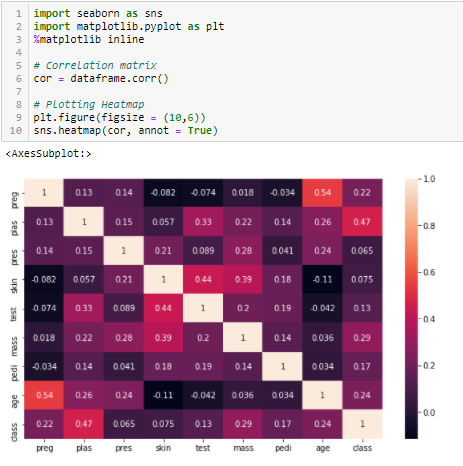We need to set an absolute value, say 0.5 as the threshold for selecting the variables. If we find that the predictor variables are correlated among themselves, we can drop the variable which has a lower correlation coefficient value with the target variable. We can also compute multiple correlation coefficients to check whether more than two variables are correlated to each other. This phenomenon is known as multicollinearity.

#### Variance Threshold

The variance threshold is a simple baseline approach to feature selection. It removes all features which variance doesn’t meet some threshold. By default, it removes all zero-variance features, i.e., features that have the same value in all samples. We assume that features with a higher variance may contain more useful information, but note that we are not taking the relationship between feature variables or feature and target variables into account, which is one of the drawbacks of filter methods.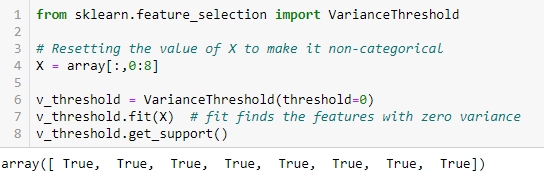The get_support returns a Boolean vector where True means that the variable does not have zero variance.

‘The mean absolute difference (MAD) computes the absolute difference from the mean value. The main difference between the variance and MAD measures is the absence of the square in the latter. The MAD, like the variance, is also a scale variant.’  This means that higher the MAD, higher the discriminatory power.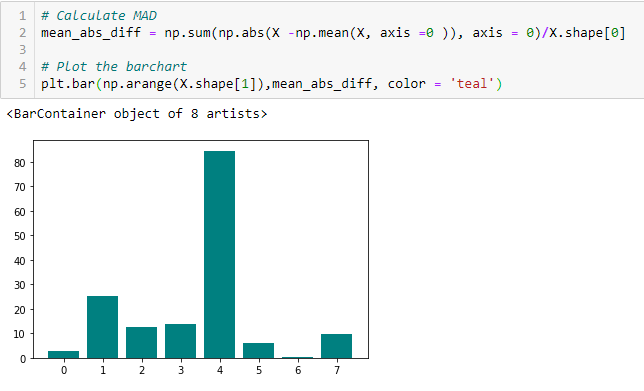#### Dispersion ratio

‘Another measure of dispersion applies the arithmetic mean (AM) and the geometric mean (GM). For a given (positive) feature Xi on n patterns, the AM and GM are given by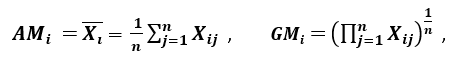respectively; since AMi ≥ GMi, with equality holding if and only if Xi1 = Xi2 = …. = Xin, then the ratio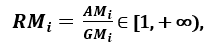can be used as a dispersion measure. Higher dispersion implies a higher value of Ri, thus a more relevant feature. Conversely, when all the feature samples have (roughly) the same value, Ri is close to 1, indicating a low relevance feature.’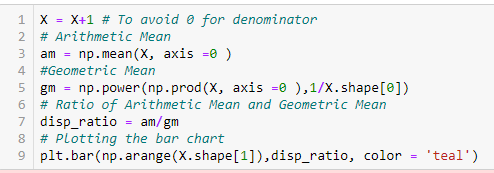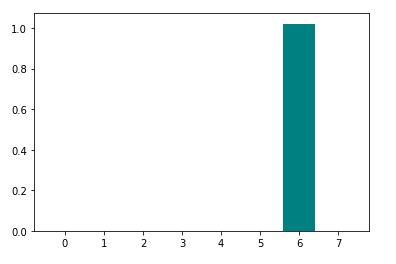### B. Wrapper Methods:

Wrappers require some method to search the space of all possible subsets of features, assessing their quality by learning and evaluating a classifier with that feature subset. The feature selection process is based on a specific machine learning algorithm that we are trying to fit on a given dataset. It follows a greedy search approach by evaluating all the possible combinations of features against the evaluation criterion. The wrapper methods usually result in better predictive accuracy than filter methods.

Let’s, discuss some of these techniques:

#### Forward Feature Selection

This is an iterative method wherein we start with the best performing variable against the target. Next, we select another variable that gives the best performance in combination with the first selected variable. This process continues until the preset criterion is achieved.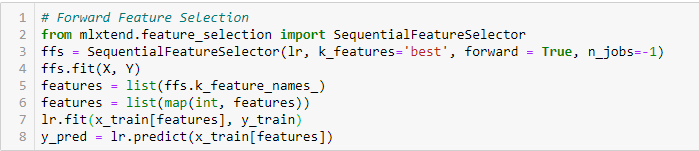#### Backward Feature Elimination

This method works exactly opposite to the Forward Feature Selection method. Here, we start with all the features available and build a model. Next, we the variable from the model which gives the best evaluation measure value. This process is continued until the preset criterion is achieved.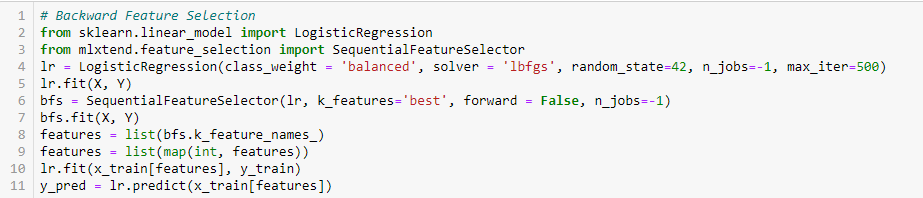This method along with the one discussed above is also known as the Sequential Feature Selection method.

#### Exhaustive Feature Selection

This is the most robust feature selection method covered so far. This is a brute-force evaluation of each feature subset. This means that it tries every possible combination of the variables and returns the best performing subset.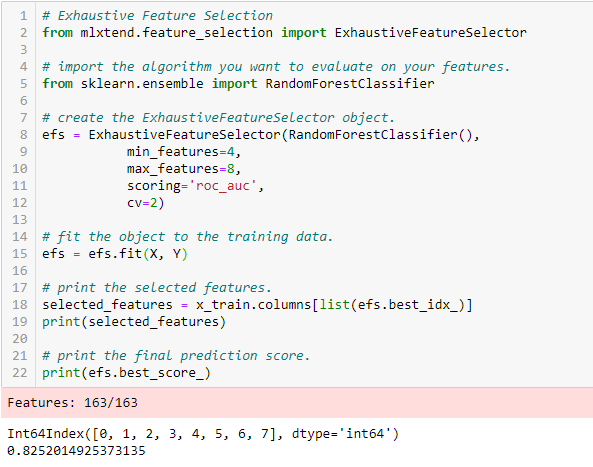#### Recursive Feature Elimination

Given an external estimator that assigns weights to features (e.g., the coefficients of a linear model), the goal of recursive feature elimination (RFE) is to select features by recursively considering smaller and smaller sets of features. First, the estimator is trained on the initial set of features and the importance of each feature is obtained either through a coef_ attribute or through a feature_importances_ attribute.

Then, the least important features are pruned from the current set of features. That procedure is recursively repeated on the pruned set until the desired number of features to select is eventually reached.’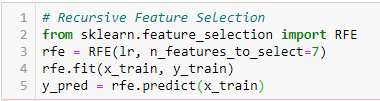### C. Embedded Methods:

These methods encompass the benefits of both the wrapper and filter methods, by including interactions of features but also maintaining reasonable computational cost. Embedded methods are iterative in the sense that takes care of each iteration of the model training process and carefully extracts those features which contribute the most to the training for a particular iteration.

#### LASSO Regularization (L1)

Regularization consists of adding a penalty to the different parameters of the machine learning model to reduce the freedom of the model, i.e. to avoid over-fitting. In linear model regularization, the penalty is applied over the coefficients that multiply each of the predictors. From the different types of regularization, Lasso or L1 has the property that is able to shrink some of the coefficients to zero. Therefore, that feature can be removed from the model.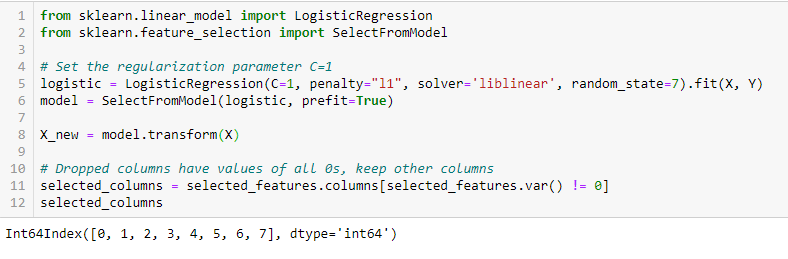#### Random Forest Importance

Random Forests is a kind of a Bagging Algorithm that aggregates a specified number of decision trees. The tree-based strategies used by random forests naturally rank by how well they improve the purity of the node, or in other words a decrease in the impurity (Gini impurity) over all trees. Nodes with the greatest decrease in impurity happen at the start of the trees, while notes with the least decrease in impurity occur at the end of trees. Thus, by pruning trees below a particular node, we can create a subset of the most important features.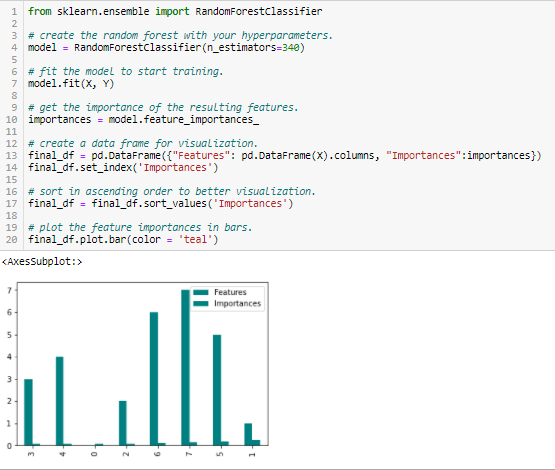## Conclusion

We have discussed a few techniques for feature selection. We have on purpose left the feature extraction techniques like Principal Component Analysis, Singular Value Decomposition, Linear Discriminant Analysis, etc. These methods help to reduce the dimensionality of the data or reduce the number of variables while preserving the variance of the data.

Apart from the methods discussed above, there are many other methods of feature selection. There are hybrid methods too that use both filtering and wrapping techniques. If you wish to explore more about feature selection techniques, great comprehensive reading material in my opinion would be ‘Feature Selection for Data and Pattern Recognition’ by Urszula Stańczyk and Lakhmi C. Jain.

## References

Paper named ‘Efficient feature selection filters for high-dimensional data’ by Artur J. Ferreira , Mário A.T. Figueiredo